Physics Letters A
Volume 372, Issue 24, 9 June 2008, Pages 4344-4349

Tachyonic γ-ray spectra of active galactic nuclei: Evidence for intrinsic spectral curvature

aDepartment of Physics, Hiroshima University, 1-3-1 Kagami-yama, Higashi-Hiroshima 739-8526, Japan

revised 15 April 2008;
accepted 15 April 2008.
Communicated by V.M. Agranovich.
Available online 18 April 2008.

### Abstract

Tachyonic spectral densities of ultra-relativistic electron populations are fitted to the γ-ray spectra of two TeV blazars, the BL Lacertae objects 1ES 0229+200 and 1ES 0347-121. The spectral maps are compared to Galactic TeV sources, the γ-ray binary LS 5039 and the supernova remnant W28. In contrast to TeV photons, the extragalactic tachyon flux is not attenuated by interaction with the cosmic background light; there is no absorption of tachyonic γ-rays via pair creation, as tachyons do not interact with infrared background photons. The curvature of the observed γ-ray spectra is intrinsic, caused by the Boltzmann factor of the electron densities, and reproduced by a tachyonic cascade fit. In particular, the curvature in the spectral map of the Galactic microquasar is more pronounced than of the two extragalactic γ-ray sources. Estimates of the thermodynamic parameters of the thermal or, in the case of supernova remnant W28, shock-heated nonthermal electron plasma generating the tachyon flux are obtained from the spectral fits.

Keywords: Superluminal radiation; Tachyonic cascade spectra; Ultra-relativistic electron plasma; TeV blazars; Spectral averaging; Proca equation with negative mass-square

PACS classification codes: 03.50.Kk; 05.30.Fk; 52.27.Ny; 95.30.Gv

### 1. Introduction

The goal is to point out evidence for superluminal γ-rays from two active galactic nuclei, the BL Lacertae objects 1ES 0229+200, cf. Refs. , ,  and , and 1ES 0347-121, cf. Refs. ,  and . Spectral maps of these TeV blazars have recently been obtained by means of ground-based imaging air Cherenkov detectors  and . Here, a tachyonic cascade fit is performed to the γ-ray spectrum of these blazars. In contrast to electromagnetic γ-rays, tachyons cannot interact with infrared background photons, so that there is no attenuation of the extragalactic tachyon flux by electron–positron pair production. The observed spectrum is already the intrinsic one without any need of absorption correction as required in electromagnetic spectral fits  and . The spectral curvature is generated by the Boltzmann factor of the thermal electron plasma in the galactic nuclei.

Tachyonic cascade spectra are obtained by averaging the superluminal spectral densities of individual electrons with ultra-relativistic Fermi distributions. We use the averaged radiation densities to perform spectral fits to the γ-ray spectra of the mentioned BL Lacertae objects as well as the microquasar LS 5039 and the supernova remnant W28. Tachyonic cascade spectra generated by thermal electron populations in the active galactic nuclei (AGNs) provide excellent fits to the observed γ-ray flux. The temperature, source count, and internal energy of the ultra-relativistic electron plasma are obtained from the spectral fits. The spectral map of the Galactic microquasar is no less curved than the cascades in the AGN spectra. Moreover, the spectral curvature of the AGNs does not increase with increasing redshift, which is further evidence for an unattenuated extragalactic γ-ray flux.

The tachyonic radiation field is a Proca field with negative mass-square,

coupled to an electron current, where mt is the mass of the superluminal Proca field Aα, and q the tachyonic charge carried by the subluminal current ,  and . The mass term in (1) is added with a positive sign, and the sign convention for the metric is diag(−1,1,1,1), so that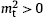is the negative mass-square of the radiation field. The sign convention for the field tensor is Fμν=Aν,μAμ,ν. The negative mass-square refers to the radiation field rather than the current, in contrast to the traditional approach based on superluminal source particles emitting electromagnetic radiation  and . The superluminal radiation field does not carry any kind of charge, tachyonic charge q is a property of subluminal particles, as is electric charge . The field equations derived from (1) read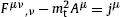, and can equivalently be written as Proca equation,, subject to the Lorentz condition, which follows from current conservation. An estimate of the tachyon–electron mass ratio obtained from hydrogenic Lamb shifts is mt/m≈1/238 .

In Section 2, we further elaborate on the tachyonic Proca equation by comparing to electromagnetic theory, and assemble the tachyonic spectral averages employed in the fits, which are based on the transversal and longitudinal radiation densities generated by a free electronic spinor current. In Section 3, the spectral fitting is explained, and intrinsic spectral curvature is argued by comparing the cascade spectra of the above-mentioned Galactic and extragalactic TeV sources. The conclusions are summarized in Section 4.

### 2. Spectral maps in the γ-ray bands: Origin and structure of tachyonic cascade spectra

The Lagrangian (1) resembles its electrodynamic counterpart, but the negative mass-square of the Proca field causes striking differences. Apart from the superluminal speed of the tachyonic quanta, the radiation is partially longitudinally polarized , the gauge freedom is broken, and freely propagating charges can radiate superluminal quanta. The analogy to Maxwell's theory becomes even more transparent in 3D. The tachyonic E and B fields are related to the vector potential Aα=(A0,A) by E=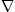A0−∂A/∂t and B=rotA, and the field equations decompose into

where we identified jμ=(ρ,j). The vector potential is completely determined by the current and the field strengths; there is no gauge freedom owing to the tachyon mass. Another major difference to electromagnetic theory is the emergence of longitudinal wave propagation. To see this, we consider monochromatic waves,, and analogously for the scalar potential and the field strengths, with amplitudes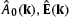, and, respectively. We write k=k(ω)k0, with a unit wave vector k0. On substituting this ansatz into the free field equations (2), we find the dispersion relation. The transversality condition on the vector potential is, so that the remaining transversal amplitudes are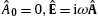, and. If the product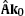does not vanish, the modes must be longitudinal, satisfying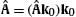. In this case,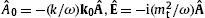, and, so that a longitudinal plane wave has no magnetic component.

The spectral fits in Section 3 are based on the quantized tachyonic radiation densities

generated by the spinor currentof a uniformly moving electron . The superscripts T and L refer to the transversal/longitudinal polarization components defined by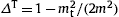and ΔL=0. γ is the electronic Lorentz factor, αq the tachyonic fine structure constant, and mt the tachyon mass. In the γ-ray broadband fit of supernova remnant W28, the radiation frequencies ω stretch over five decades, cf. Section 3. The units=c=1 can easily be restored. We use the Heaviside–Lorentz system, so that αq=q2/(4πc)≈1.0×10−13 and mt≈2.15 keV/c2; the tachyon–electron mass ratio is mt/m≈1/238. These estimates are obtained from Lamb shifts of hydrogenic ions . A spectral cutoff occurs at

Only frequencies in the range 0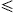ωωmax(γ) can be radiated by a uniformly moving electron, the tachyonic spectral densities pT,L(ω,γ) being cut off at the break frequency ωmax. A positive ωmax(γ) requires Lorentz factors exceeding the threshold μt in (4), since ωmax(μt)=0. The lower threshold on the speed of the electron for radiation to occur is thus υmin=mt/(2mμt). The tachyon–electron mass ratio gives υmin/c≈2.1×10−3, which is roughly the speed of the Galaxy in the microwave background .

The radiation densities (3) refer to a single spinning charge with Lorentz factor γ; we average them with a Fermi power-law distribution  and ,

The particle number is found as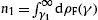, where γ1 is the lower edge of Lorentz factors in the source population. m is the electron mass, and the exponential cutoff is related to the electron temperature by β=m/(kT). The power-law exponent δ ranges in a narrow interval in astrophysical spectral averages , , , , , , ,  and , which are usually done in the classical limit (13) with 0δ4. Otherwise, there are no conceptual constraints on the power-law index of these stationary non-equilibrium distributions. Thermal equilibrium is recovered with δ=0 and γ1=1; the spectral fits of the blazars and the microquasar in Fig. 1, Fig. 2 and Fig. 3 are performed with equilibrium distributions. The shocked electron plasma of supernova remnant W28 requires δ≈3.6 to fit the steep power-law slope in Fig. 4. The exponent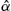in (5) defines the fugacity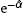; we use a hat to distinguishfrom the electron index customarily defined as α=δ−2, cf. (13). The fugacity is related to the chemical potential μ by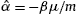.Fig. 1. Spectral map of the BL Lac 1ES 0229+200. HESS data points from . The solid line T+L depicts the unpolarized differential tachyon flux dNT+L/dE, obtained by adding the flux densities ρ1,2 of two electron populations, cf. (16) and Table 1. The transversal (T, dot-dashed) and longitudinal (L, double-dot-dashed) flux densities add up to the total unpolarized flux T+L. The exponential decay of the cascades ρ1,2 sets in at about Ecut≈(mt/m)kT, implying cutoffs at 3.6 TeV for the ρ1 cascade and 120 GeV for ρ2.Fig. 2. Spectral map of the BL Lac 1ES 0347-121. HESS points from . The unpolarized spectral fit T+L is based on the electron distributions recorded in Table 1, the polarized flux components are labeled T and L. The ρ1 cascade is cut at Ecut≈4.0 TeV, and ρ2 at 190 GeV. The curvature in the spectral slope of 1ES 0347-121 at z≈0.188 is less pronounced than of 1ES 0229+200 at z≈0.140, suggesting that the shape of the rescaled flux densityis intrinsic rather than generated by intergalactic absorption.Fig. 3. Spectral map of the γ-ray binary LS 5039 close to periastron. HESS data points at the superior conjunction . Notation as in Fig. 1. The cascade ρ1 is exponentially cut at Ecut≈6.3 TeV, and ρ2 at 190 GeV, cf. Table 1. The spectral map of this microquasar at a distance of 2.5 kpc is more strongly curved than of the AGNs in Fig. 1 and Fig. 2, indicating that the curvature of the AGN spectra is intrinsic as well, generated by the Boltzmann factor of the thermal electron populations, cf. caption to Fig. 2.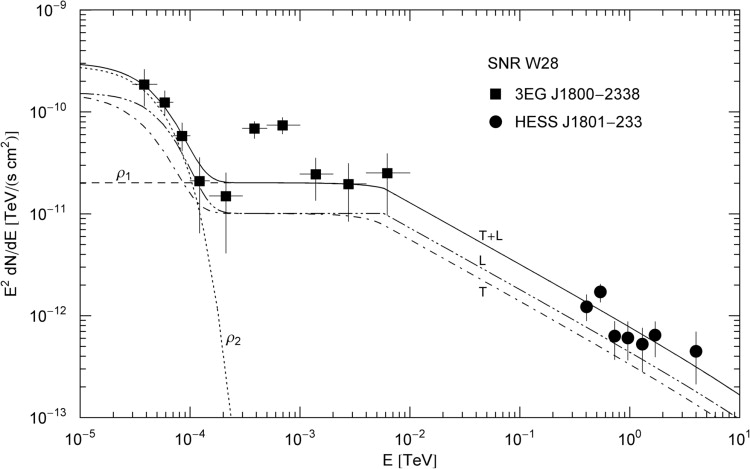Fig. 4. γ-Ray broadband of the TeV source HESS J1801-233 and the associated EGRET source 3EG J1800-2338 at the northeast boundary of supernova remnant W28. Data points from , also see  and . Notation as in Fig. 1. The nonthermal cascade ρ1 admits a power-law slope E1−α, α≈1.6, adjacent to the MeV–GeV plateau typical for tachyonic cascade spectra  and . A spectral break at mtγ1≈5.8 GeV is visible as edge in the longitudinal component. The curvature of the thermal cascade ρ2 in the MeV range is due to the exponential cutoff at (mt/m)kT≈20 MeV, cf. Table 1.

The spectral average of the radiation densities (3) is carried out as

where θ is the Heaviside step function. The spectral range of densities (3) is bounded by ωmax in (4), so that the solution of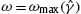defines the minimal electronic Lorentz factor for radiation at this frequency ,

The average (6) can be reduced to the fermionic spectral functions

with lower integration boundary γ1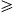μt, cf. after (4). This threshold Lorentz factor γ1 defines the break frequency,

which separates the spectrum into a low- and high-frequency band . By making use of the spectral functions (8), we can write the averaged radiation densities (6) as

with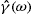in (7) and ω1 in (9), so that. The superscripts T and L denote the transversal and longitudinal radiation components, cf. (3). The spectral functions FT,L(ω,γ1) in (10) are obtained by substituting radiation densities (3) into the integral representation (8),

where the weight factors fk read

with density dρF(γ) in (5).

The quasiclassical fugacity expansion of the spectral functions (11) is found by expanding density (5) in ascending powers of. In leading order, dρF(γ)dρα,β(γ),

This power-law density is the classical limit of the fermionic density dρF. As mentioned above, exponentin the normalization factor Aα,β defines the fugacity, and is not to be confused with the electron index α=δ−2 in (13). The leading order of the reduced spectral functions (12) can be written as incomplete Γ-function, fk(γ1)Aα,ββδkΓ(kδ,βγ1), obtained by replacing the fermionic dρF in the weights (12) by the Boltzmann power-law density (13).

The classical limit of the fermionic spectral functions FT,L(ω,γ1) in (8) is the Boltzmann average 

Here,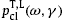is the classical tachyonic spectral density, recovered by dropping all terms containing mt/m ratios in (3) and (4). In particular,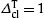and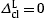, cf. (3). The classical spectral functions BT,L(ω,γ1) are obtained from the Fermi functions FT,L(ω,γ1) in (11) by dropping the mt/m terms, and substituting the leading order of the fugacity expansion of the weights fk(γ1) as stated after (13). The classical limit of the fermionic spectral averagepT,L(ω)F in (10) thus reads

whereis the classical limit of the break frequency (9).

### 3. Spectral curvature of Galactic and extragalactic TeV γ-ray sources: Does distance matter?

The spectral fits of the active galactic nuclei (AGNs) and the Galactic TeV sources in Fig. 1, Fig. 2, Fig. 3 and Fig. 4 are based on the E2-rescaled flux densities

where d is the distance to the source andpT,L(ω)α,β the spectral average (15) (with ω=E/). The fits are done with the unpolarized flux density dNT+L=dNT+dNL of two electron populations ρi=1,2, cf. Table 1. Each electron density generates a cascade ρi, and the wideband comprises two cascade spectra labeled ρ1 and ρ2 in the figures. As for the electron count,, we use a rescaled parameter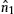for the fit,

which is independent of the distance estimate in (16). Here,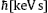implies the tachyon mass in keV units, that is, we put mt≈2.15 in the spectral densities (3). At γ-ray energies, only a tiny αq/αe-fraction (the ratio of tachyonic and electric fine structure constants) of the tachyon flux is absorbed by the detector, which requires a rescaling of the electron count n1, so that the actual number of radiating electrons is, cf. Ref. . We thus find the electron count as, wheredefines the tachyonic flux amplitude extracted from the spectral fit. (In Table 1, the subscript 1 ofandhas been dropped.) Electron temperature and cutoff parameter in the Boltzmann factor of density (13) are related by kT[TeV]≈5.11×10−7/β, and the energy estimates of the thermal cascades in Table 1 are based on, cf. Ref. . (The renormalized countis to be identified with the particle number N in the thermodynamic functions discussed in this reference.) The distance estimates of the AGNs are based on dcz/H0, with the Hubble distance c/H0≈4.4×103 Mpc (that is, h0≈0.68). Hence, d[Mpc]≈4.4×103z, and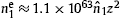, cf. Table 1.

Table 1.

Electronic source distributions ρi generating the tachyonic cascade spectra of the active galactic nuclei in Fig. 1 and Fig. 2, the microquasar LS 5039 in Fig. 3, and the supernova remnant W28 in Fig. 4. Each ρi stands for a thermal Maxwell–Boltzmann density dρα=−2,β(γ) with γ1=1, apart from the ρ1 distribution of SNR W28, which is a power-law density with α≈1.6 and γ1≈2.7×106, cf. (13) and after (5). β is the cutoff parameter in the Boltzmann factor.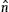determines the amplitude of the tachyon flux generated by the electron density ρi, from which the electron count ne is inferred at the indicated distance d, cf. after (17). kT is the temperature and U the internal energy of the electron populations, cf. after (16). The parameters β andare extracted from the least-squares fit T+L in Fig. 1, Fig. 2, Fig. 3 and Fig. 4

βd ne kT (TeV) U (erg)
1ES 0229+200 z≈0.140
ρ1 5.97×10−10 6.6×10−5 620 Mpc 1.4×1057 860 5.8×1060
ρ2 1.79×10−8 7.5×10−4 1.6×1058 29 2.2×1060
1ES 0347-121 z≈0.188
ρ1 5.38×10−10 2.6×10−5 830 Mpc 1.0×1057 950 4.6×1060
ρ2 1.13×10−8 2.3×10−4 8.9×1057 45 1.9×1060
LS 5039 (sup. conj.) 2.5 kpc
ρ1 3.36×10−10 6.4×10−5 2.3×1046 1500 1.7×1050
ρ2 1.13×10−8 2.2×10−4 7.9×1046 45 1.7×1049
SNR W28 1.9 kpc
ρ1 2.2×10−3 4.6×1047
ρ2 1.08×10−4 3.1×10−2 6.4×1048 4.7×10−3 1.5×1047

Fig. 1 shows the tachyonic spectral map of the BL Lacertae object (BL Lac) 1ES 0229+200, located at a redshift of z≈0.140, cf. Refs. , ,  and . The flux points were obtained with the HESS array of atmospheric Cherenkov telescopes in the Khomas Highland of Namibia . The χ2-fit is done with the unpolarized tachyon flux T+L, and subsequently split into transversal and longitudinal components. The differential flux is rescaled with E2 for better visibility of the spectral curvature. Temperature and source count of the electron populations generating the cascades are recorded in Table 1. In Fig. 2, we show the spectral map of the BL Lac 1ES 0347-121, at a redshift of z≈0.188, cf. Refs. ,  and . TeV γ-ray spectra of BL Lacs are usually assumed to be generated by inverse Compton scattering or proton–proton scattering followed by pion decay . Both mechanisms result in a flux of TeV photons, assumed to be partially absorbed by interaction with infrared background photons owing to pair creation, so that the intrinsic spectrum has to be reconstructed on the basis of intergalactic absorption models depending on vaguely known cosmological input parameters . The extragalactic tachyon flux is not attenuated by interaction with the background light, there is no absorption of tachyonic γ-rays. The superluminal Proca field (1) is minimally coupled to the electron current, and does not directly interact with electromagnetic radiation.

The spectral curvature apparent in double-logarithmic plots of the E2-rescaled flux densities (16) is intrinsic, caused by the Boltzmann factor of the electron populations generating the tachyon flux. The curvature present in TeV γ-ray spectra does not increase with distance, at least there is no evidence to that effect if we compare the spectral slopes of the blazars in Fig. 1 and Fig. 2, and the spectral maps of other flaring AGNs such as H1426+428 at z≈0.129 and 1ES 1959+650 at z≈0.047, cf. Ref. . Further evidence for intrinsic spectral curvature is provided by Fig. 3, depicting the spectral map of the microquasar LS 5039, a compact object orbiting a massive O-star ,  and . The spectral curvature of this Galactic binary is even more pronounced than of the BL Lacs in Fig. 1 and Fig. 2. The same holds true for the HESS spectral map of LS 5039 at the inferior conjunction studied in Ref. .

Fig. 4 depicts the γ-ray wideband of the TeV source HESS J1801-233 and the coincident EGRET point source 3EG J1800-2338 ,  and . The extended TeV source is located on the northeastern rim of the supernova remnant (SNR) W28, a mixed-morphology SNR interacting with molecular clouds  and . The spectral map of SNR W28 in Fig. 4 is to be compared to the unpulsed γ-ray spectrum of the Crab Nebula, cf. Fig. 1 in Ref. , the spectral map of SNR RX J1713.7-3946 in Fig. 2 of Ref. , and in particular to the unidentified TeV source TeV J2032+4130 in conjunction with the associated EGRET source 3EG J2033+4118, cf. Fig. 6 of Ref. . A spectral plateau in the MeV to GeV range occurs frequently in spectral maps of TeV γ-ray sources, and can easily be fitted with tachyonic cascade spectra, in contrast to electromagnetic inverse-Compton fits. SNR W28 is located at a distance of 1.9 kpc . The thermal cascade in the MeV range (ρ2 in Fig. 4) preceding the spectral plateau is strongly curved; the power-law slope of the ρ1 cascade also terminates in exponential decay, but outside the presently accessible TeV range shown in the figure. The cutoff temperature of the nonthermal electron density generating cascade ρ1 is too high to bend the power-law slope in the TeV range covered in Fig. 4, so that the internal energy of the shock-heated plasma could not be determined from the χ2-fit, in contrast with the power-law index α and the threshold Lorentz factor γ1, cf. caption to Table 1.

### 4. Conclusion

The spectral maps of two TeV γ-ray blazars have been fitted with tachyonic cascade spectra and compared to a Galactic γ-ray binary and supernova remnant. Table 1 contains estimates of the thermodynamic parameters of the electron populations generating the superluminal cascades. The spectral curvature is intrinsic and reproduced by the tachyonic spectral densities (3) averaged with ultra-relativistic thermal electron distributions, cf. Fig. 1, Fig. 2 and Fig. 3. The shocked electron plasma in SNR W28 requires a nonthermal power-law distribution to adequately reproduce the TeV cascade in Fig. 4. The curvature in the γ-ray spectra of BL Lacs is uncorrelated with distance, so that absorption of electromagnetic radiation due to interaction with infrared photons is not an attractive explanation of spectral curvature. By contrast, there is no attenuation of the extragalactic tachyon flux, as tachyons cannot interact with cosmic background photons, so that the observed cascades are the intrinsic spectrum. The distance of the AGNs does not show in the spectral curvature, as tachyonic cascades are unaffected by background photons.

### Acknowledgements

The author acknowledges the support of the Japan Society for the Promotion of Science. The hospitality and stimulating atmosphere of the Centre for Nonlinear Dynamics, Bharathidasan University, Trichy, and the Institute of Mathematical Sciences, Chennai, are likewise gratefully acknowledged.

### References

 R. Falomo and J.K. Kotilainen, Astron. Astrophys. 352 (1999), p. 85.

 D. Horan et al., Astrophys. J. 603 (2004), p. 51.

 D.A. Williams, AIP Conf. Proc. 745 (2005), p. 499.

 F. Aharonian et al., Astron. Astrophys. 475 (2007), p. L9.

 C.M. Urry et al., Astrophys. J. 532 (2000), p. 816.

 M. Giroletti et al., Astrophys. J. 613 (2004), p. 752.

 F. Aharonian et al., Astron. Astrophys. 473 (2007), p. L25.

 K. Tsuchiya et al., Astrophys. J. 606 (2004), p. L115.

 J. Albert et al., Astrophys. J. 662 (2007), p. 892.

 R. Tomaschitz, Eur. Phys. J. D 32 (2005), p. 241.

 R. Tomaschitz, Eur. Phys. J. C 49 (2007), p. 815.

 R. Tomaschitz, Ann. Phys. 322 (2007), p. 677.

 S. Tanaka, Prog. Theor. Phys. 24 (1960), p. 171.

 Ya.P. Terletsky, Sov. Phys. Dokl. 5 (1961), p. 782.

 R. Tomaschitz, Class. Quantum Grav. 18 (2001), p. 4395.

 R. Tomaschitz, Eur. Phys. J. B 17 (2000), p. 523.

 W.-M. Yao et al., J. Phys. G 33 (2006), p. 1.

 R. Tomaschitz, Physica A 385 (2007), p. 558.

 R. Tomaschitz, Physica A 387 (2008), p. 3480.

 S.P. Reynolds and J.W. Keohane, Astrophys. J. 525 (1999), p. 368.

 R. Enomoto et al., Astrophys. J. 652 (2006), p. 1268.

 T. Nakamori et al., Astrophys. J. 677 (2008), p. 297.

 J.M. Fierro et al., Astrophys. J. 494 (1998), p. 734.

 R. Tomaschitz, Astropart. Phys. 23 (2005), p. 117.

 R. Tomaschitz, Astropart. Phys. 27 (2007), p. 92.

 R. Tomaschitz, Physica A 335 (2004), p. 577.

 I. de Pater and B.J. Butler, Icarus 163 (2003), p. 428.

 I. de Pater et al., Icarus 163 (2003), p. 434.

 R. Tomaschitz, Physica A 320 (2003), p. 329.

 R. Tomaschitz, Eur. Phys. J. C 45 (2006), p. 493.

 R. Tomaschitz, Phys. Lett. A 366 (2007), p. 289.

 F. Aharonian et al., Astron. Astrophys. 460 (2006), p. 743.

 M.V. McSwain et al., Astrophys. J. 600 (2004), p. 927.

 J. Casares et al., Mon. Not. R. Astron. Soc. 364 (2005), p. 899.

 F. Aharonian et al., Astron. Astrophys. 481 (2008), p. 401.

 R.C. Hartman et al., Astrophys. J. Suppl. 123 (1999), p. 79.

 L. Zhang and K.S. Cheng, Astron. Astrophys. 335 (1998), p. 234.

 P.F. Velázquez et al., Astron. J. 124 (2002), p. 2145.

 J. Rho and K.J. Borkowski, Astrophys. J. 575 (2002), p. 201.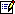Tel.: +81 824 247361; fax: +81 824 240717.